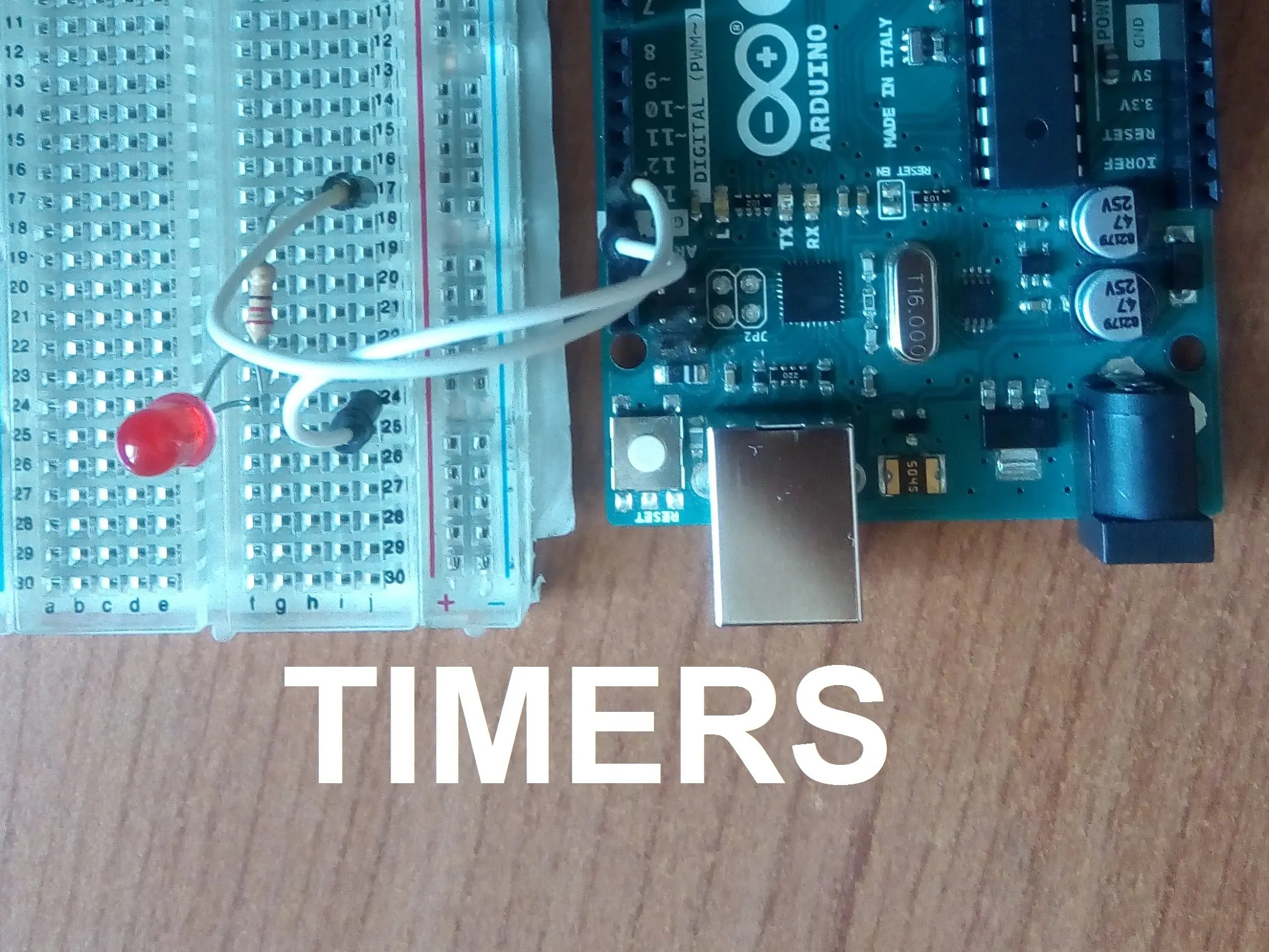Project tutorialInternal Timers of Arduino © GPL3+

In this tutorial I will explain how to use the TIMER0 of Arduino

• 10,960 views
• 44 respects

Components and supplies

Overview

If you need to count accurate time you need to use a timer, but usually it isn't so easy to use the internal timers of Arduino, so in this tutorial I try to explain how to use them in an easy way.

It is so important to use timers because during the delay() function you can't do anything, but with a timer you can do everything because when the moment does arrive, it activates the interrupt.

I use the TIMER0 because it is the easiest timer, maybe in the future I may explain the other timers.

How It Works

The first thing you need to see is the datasheet of ATmega328P.

This is the counter block diagram, and looking at this you can understand how it works.

The prescaler receives a pulse from a clock cycle and then passes it to the Control Logic, therefore the Control Logic increments the TCNTn register by 1.

Now we can compare the value of TCNTn with a specific value. When the TCNTn register arrives at this value, you know that it is passed a specific time.

This method is called CTC mode for "Clear Timer on Compare". The value TCNTn register is compared to the OCRn register, when a compare match occurs the TOVn generates an interrupt.

Another important thing is the prescaler, with this you can create different divisions of the clock, in fact 16MHz is too much but, thanks to the prescaler, you can create some submultiples of it. This depends on the configuration of the TCCR0B register.

Another important thing is determine the value of OCRn register to count a specific time, you need a bit of math.

I know that all of this can seem too complicated but I'm sure that the code below will clear up each question.

In this case, we activate an interrupt request each 0.001 seconds.

TCCR0A|=(1<<WGM01);    //Set the CTC mode
OCR0A=0xF9;            //Set the value for 1ms
TIMSK0|=(1<<OCIE0A);   //Set the interrupt request
sei();                 //Enable interrupt
TCCR0B|=(1<<CS01);    //Set the prescale 1/64 clock
TCCR0B|=(1<<CS00);
ISR(TIMER0_COMPA_vect){    //This is the interrupt request
}

It is important to set the prescaler at the end because after this instruction the timer starts to count, if you need to stop it, you must reset all the bits of TCCR0B.

Below there is an example.

Code

Internal timerArduino
/*
This program turns on and off a LED on pin 13 each 1 second using an internal timer
*/

int timer=0;
bool state=0;
void setup() {
pinMode(13,OUTPUT);

TCCR0A=(1<<WGM01);    //Set the CTC mode
OCR0A=0xF9; //Value for ORC0A for 1ms

TIMSK0|=(1<<OCIE0A);   //Set the interrupt request
sei(); //Enable interrupt

TCCR0B|=(1<<CS01);    //Set the prescale 1/64 clock
TCCR0B|=(1<<CS00);

}

void loop() {
//in this way you can count 1 second because the nterrupt request is each 1ms
if(timer>=1000){
state=!state;
timer=0;
}

digitalWrite(13,state);

}

ISR(TIMER0_COMPA_vect){    //This is the interrupt request
timer++;
}

SchematicsMagconn With Arduino Can Control Internal Environment!

Project tutorial by Team Kangwon National University ECEE

• 412 views
• 4 respects

Arduino - Web Pattern Unlock

Project tutorial by phpoc_man

• 11,304 views
• 1 comment
• 62 respects

• 3,176 views
• 1 comment
• 8 respects

Talking Clock 2 - New Version (Bilingual: EN-PT)

Project tutorial by LAGSILVA

• 7,378 views
• 33 respects

Hacking Qualcomm (Quick Charge) QC 2.0/3.0 With ATtiny85

Project tutorial by Shahariar

• 5,906 views